GFG App
Open AppBrowser
Continue

# Balanced and Unbalanced Forces

Forces are required to move, turn, shift, release, shut, drive, drag, and so on. When you throw a ball, you are exerting energy on it to propel it through the air. A push or pull is referred to as a force. Forces can cause objects to move, and they can also slow, stop, or change the direction in which they move. e.g. the force of gravity that draws all things toward the Earth’s centre.

A force has both magnitude and direction, making it a vector quantity. It is measured in the SI unit of Newtons (N) and represented by the symbol F.

Mathematically, the force can be defined as:

F = m × a

Here, F is the force acting, m is the mass and a is the acceleration of a body.

### Classification of Force

On the basis of action of the force applied on a body, the force is classified into two as:

1. Balanced Force: When an equal amount of force is applied to the body but in opposite direction then this type of force is termed as Balanced Force. When balanced force is applied to a body then the body doesn’t motion instead, distortion of the body can happen.
2. Unbalanced Force: When an unequal amount of force is applied to the body, in the same or opposite direction then this type of force is termed as Unbalanced Force. When unbalanced force is applied to a body then the body does motion and in the direction of applied greater force.

### Balanced Force

A Balanced Force is a force that occurs when two equivalent forces work in opposite directions on a body. A body in Balanced Force maintains its position, i.e., it must continue to move at the same rate and in the same direction, or it may remain stationary.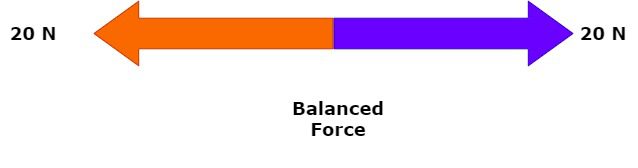If there are no forces acting on an object or balanced forces acting on it, this may happen:

• a stationary object stays at rest.
• a moving object continues to move at the same speed and in the same direction.

Keep in mind that an object will move even though no forces are acting on it.

Examples of Balanced force:

• In arm wrestling, where there is no winner, each individual exerts similar force but pushes in opposite directions. The net force (resultant force) is 0.
• If the rope does not move in a tug of war, the two teams are exerting equal yet opposing forces that are balanced. The resultant force (net force) is negative once again.
• The forces on a hanging create are equal in size but act in opposite directions. The weight pulls down and the tension in the rope pulls up.
• When the weight of an object is compensated by the upthrust from the sea, it floats. Until the weight of the water, it forces out of the way equals the weight of the object, the object will sink.
• When an object is resting on a flat surface, such as the earth, the ground’s reaction force balances the weight. The object is pushed up to the stone. When you stand still, the reaction force is what you feel in your feet. You would fall into the earth if you didn’t have this balancing force.

Types of Balanced forces:

On the basis of the direction of the force applied on the body, the balanced forces are categorized into two as:

• Internal balanced force: The type of balanced force in which the force acts in such a way that it pushes the object inwards is called the internal balanced force.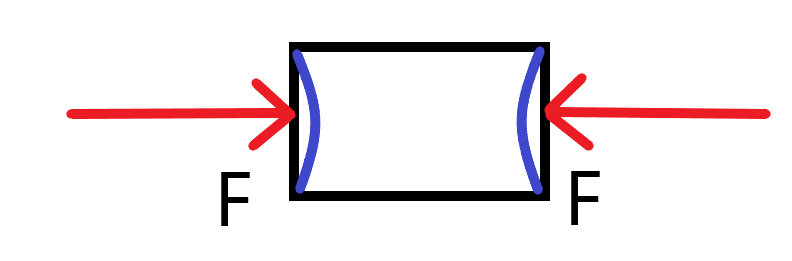Internal balanced force

• External balanced force: The type of balanced force in which the force acts in such a way that it pushes the object outwards is called the external balanced force.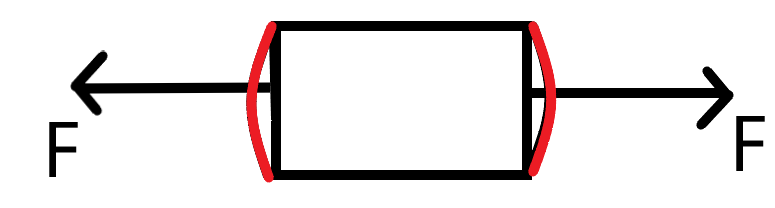### Unbalanced Force

Unbalanced force is a force that occurs when two forces acting in opposite directions on a body are not equal in magnitude and size. A body changes its position in an unbalanced force, i.e., we can see a moving object change its direction, either increasing or reducing its speed, and a body at rest begins to move, and vice versa.

When the forces on a body are unbalanced, this may happen:

• a stationary object starts to move in the direction of the resultant force.
• a moving object changes speed and direction in the direction of the resultant force.

In the example below, the resultant force is the difference between the two forces: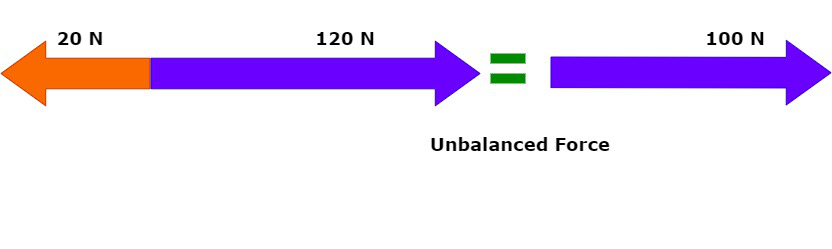Examples of Unbalanced force:

• If one side pulls harder than the other in a tug of war, the effect (net force) is that the rope changes its motion in the direction of the force with the greater strength.
• When two people pull on an object in the same direction at the same time, the object’s applied force is the result of their combined forces.
• When an apple falls from a branch, it is subjected to an unbalanced force proportional to its weight.

### Sample Problems

Problem 1: If a force of 20 N is applied to the body on the right side while a force of 20N is applied to the body on the left side and initially body is at rest. What is the behavior of the motion of the body?

Solution:

Since, the net force on the body is 0N so there is no change in the state of motion of the body, so the body
is at rest!

Problem 2: If a force of 50N is applied to the body on the right side while a force of 20N is applied to the body on the left side and initially body is at rest. What is the behavior of the motion of the body?

Solution:

As the net force on the body is 30N on the right side so there change in the state of motion of the body on the right side, so the body starts moving towards the right!

Problem 3: If a force of 50N is applied to the body on the right side while a force of 120N is applied to the body on the left side and initially body is at rest. Calculate the net force on the body and the direction of the net force.

Solution: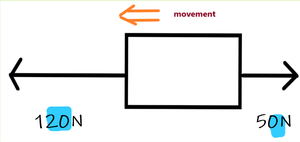Net Force = Force on left side – Force on right side

= 120N – 50N

= 70N

Direction Of Force –>  Left (As Larger Amount Of Force Is Applied On The Left Side Of The Body)

Problem 4: If the forces acting upon an object are balanced, then what happened to the object.

Solution:

The answer could be A (but does not have to be A) and it could be B (but does not have to be B). An object having balanced
forces definitely cannot be accelerating. This means that it could be at rest and staying at rest (one option) or could be in
motion at constant velocity (a second option). Either way, it definitely is not accelerating.

Problem 5: What will be the net force acting on the box shown below: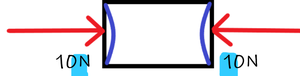Solution:

As equal forces are applied in the opposite direction so the net force is 0. So (C) is the correct answer.

My Personal Notes arrow_drop_up